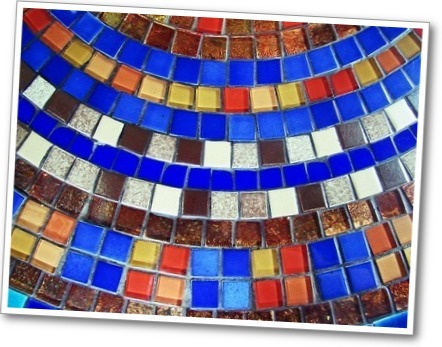# Gallery Problems# Tiling a Floor

Jack wants to tile a 12 foot by 12 foot room. He is considering two sizes of square tiles. The tiles with side length $\frac{3}{4}$ foot cost $3.89 each. The tiles with side length$1\frac{1}{3}$ foot cost$8.75 each.

1. For which size tile will the total cost of tiling the room be less? How much less will it be? Show all your work and explain how you found your answers.
2. Jack’s friend bought the $1\frac{1}{3}$ foot tiles and spent \$472.50 on the tiles. How many tiles did she buy?
1 of 5